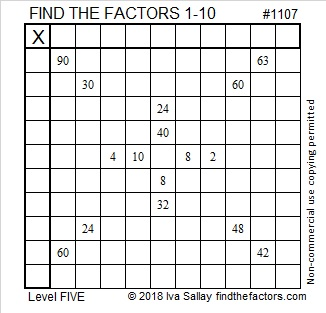# 1107 and Level 5

Some of this puzzle might be a little tricky, but you won’t allow it to trick you, right? Of course not!Print the puzzles or type the solution in this excel file: 10-factors-1102-1110

Let me tell you something about the number 1107:

• 1107 is a composite number.
• Prime factorization: 1107 = 3 × 3 × 3 × 41, which can be written 1107 = 3³ × 41
• The exponents in the prime factorization are 1 and 3. Adding one to each and multiplying we get (3 + 1)(1 + 1) = 4 × 2 = 8. Therefore 1107 has exactly 8 factors.
• Factors of 1107: 1, 3, 9, 27, 41, 123, 369, 1107
• Factor pairs: 1107 = 1 × 1107, 3 × 369, 9 × 123, or 27 × 41
• Taking the factor pair with the largest square number factor, we get √1107 = (√9)(√123) = 3√123 ≈ 33.271611107 is the hypotenuse of a Pythagorean triple:
243-1080-1107 which is 27 times (9-40-41)

This site uses Akismet to reduce spam. Learn how your comment data is processed.